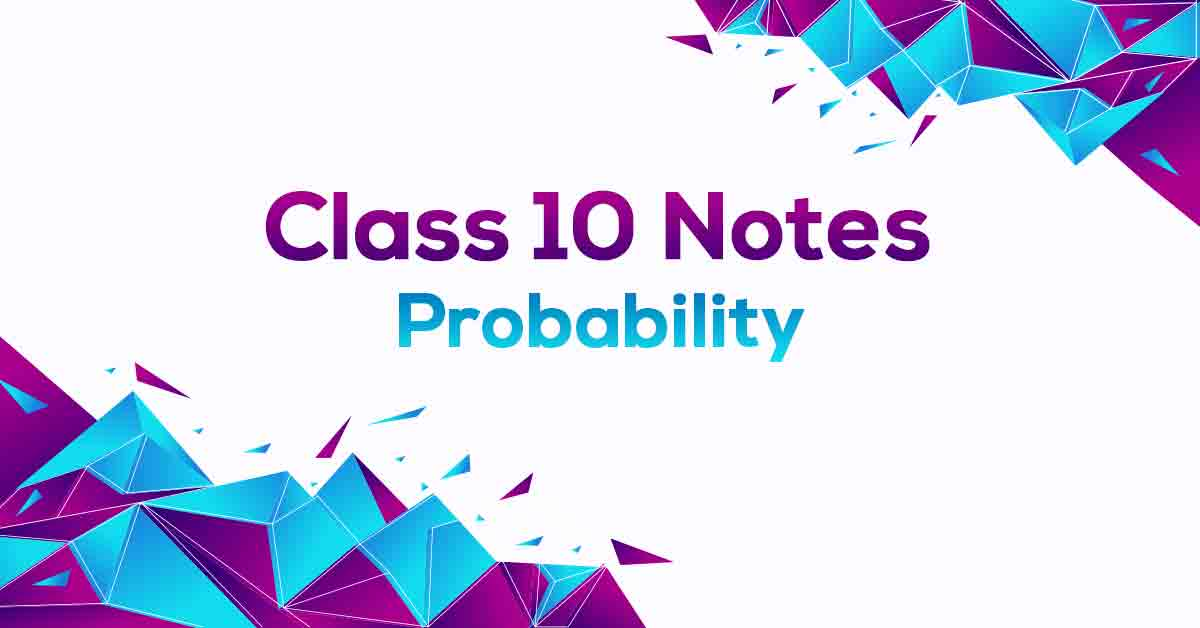Probability Class 10 Notes | Vidyakul
×

# Probability Class 10 NotesProbability Class 10 Notes are prepared in such a way that they help get rid of any confusion among children regarding the course content since CBSE keeps on updating the course every year. These notes are made highly experienced teachers strictly according to the NCERT Syllabus which offer the students a simple way to study or revise the chapter. Probability Class 10 Notes would provide you with an effective preparation for your board examinations.

### Understanding Probability.

Probability is the measure of the likeliness that an event will occur. Probability is quantified as a number between 0 and 1 (where 0 indicates impossibility and 1 indicates certainty). In mathematics, when next outcome of the experiment cannot be determined then we say it is a random experiment. A trial is an action which results in one or several outcomes, for example each toss of the coin and each throw of the die are called trials.